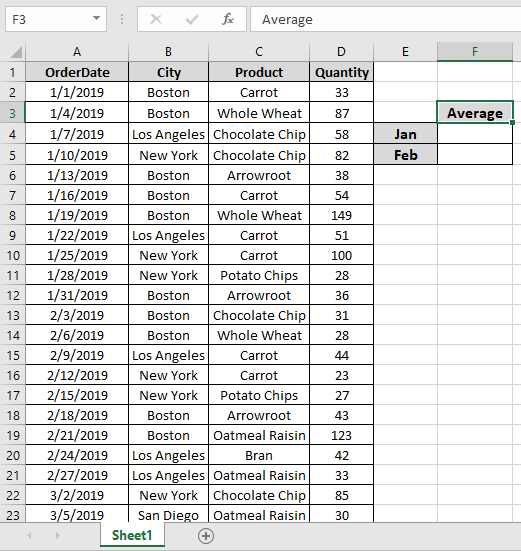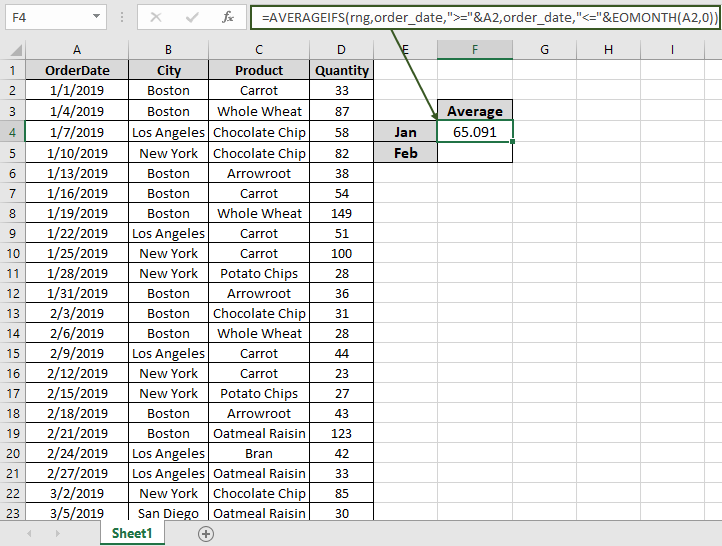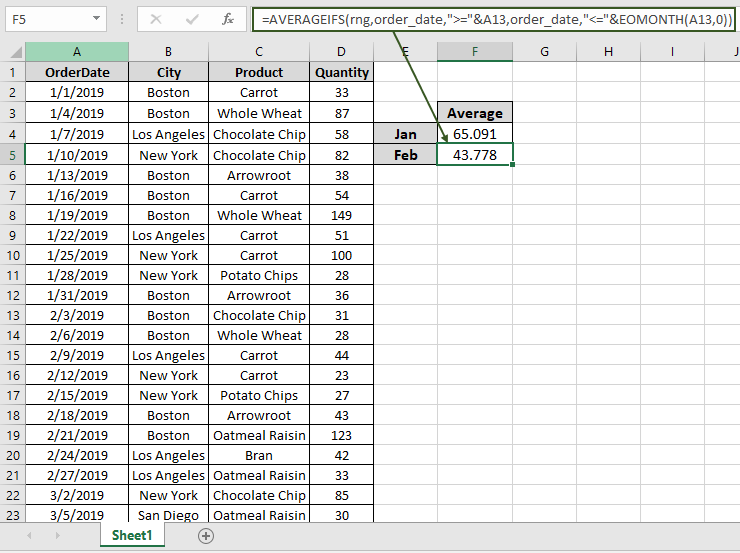How to Find Average of the Last 3 Values in Microsoft Excel 2010

In this article, we will learn How to Find the Average of the Last 3 Values in Microsoft Excel 2010.

Scenario

For Instance, you have a large list of data and you need to find the Average of the numbers from last 3 particular month.

Function and formula

For this article we will be needing the use the following functions:

1. AVERAGEIFS Function
2. EOMONTH function

Excel AVERAGEIFS function is used to find the average of the array based on multiple criteria or condition.

Syntax:

 = AVERAGEIFS ( Average_range , range1, criteria1, [ range2,criteria2 ] )

The EOMONTH function is used to find the last day of the given month. It requires two things:

1. From date you wish to count or manufacturing date.
2. Number of months from the date or its living period.

Syntax:

 =EOMONTH( Start_date, months)

start_date : a valid date representing the start date for the function.

Months : a positive or negative number to get the date for after or before the start date.

Now we will make a formula out of these functions. Here we will given the data and we needed to find the AVERAGE of the numbers in a particular month.

Use the formula:

 = AVERAGEIFS ( rng , order_date , ">=" & date , order_date , "<=" & EOMONTH ( date , 0 ) )

Explanation:

• EOMONTH function returns the end of the month date of the date given as argument.
• AVERAGEIFS takes the average of the numbers between having date greater than given date and less than the end of the month date.

Notes: do not provide date directly to the function. Use DATE function or use cell reference for date argument in excel as Excel reads date only with the correct order.

Example :

All of these might be confusing to understand. Let's understand how to use the function using an example. Here Here we have a data from A1:D51. we need to find the AVERAGE of Quantity in the month of January.First we will find a date which has the first date of the month which is the A2 cell. Named ranges are rng ( D2 : D51 ) and order_date ( A2 : A51).

Use the Formula:

 = AVERAGEIFS ( rng , order_date , ">=" & A2 , order_date , "<=" & EOMONTH ( A2 , 0 ) )

Explanation:

• EOMONTH (A2, 0) returns the 31st January as the last date in January.
• ">=" & A2 returns a criteria that the value greater than date in A2.
• "<=" & EOMONTH ( A2 , 0 ) ) returns a criteria that the value less than the end of the month date.
• AVERAGEIFS takes all the above arguments and returns the average of the number given below.

{ 33 ; 87 ; 58 ; 82 ; 38 ; 54 ; 149 ; 51 ; 100 ; 28 ; 36 }

Here the A2 is given as cell reference & Named ranges given as rng ( D2 : D51 ) and order_date ( A2 : A51).As you can see in the above snapshot the AVERAGE of the quantity in January comes out to be 65.091 .

Now we will get the AVERAGE of the quantity in February by changing the First date argument of the function

Use the Formula:

 = AVERAGEIFS ( rng , order_date , ">=" & A13 , order_date , "<=" & EOMONTH ( A13 , 0 ) )As you can see in the above snapshot the AVERAGE of the quantity in January comes out to be 43.778 .

Notes:

1.  The function returns #VALUE! error if the argument to the function is non-numeric.
2. Do not provide date directly to the function. Use DATE function or use cell reference for date argument in excel as Excel reads date only with the correct order.

Hope this article about How to Find Average of the Last 3 Values in Microsoft Excel 2010 is explanatory. Find more articles on extracting values and related Excel formulas here. If you liked our blogs, share it with your friends on Facebook. And also you can follow us on Twitter and Facebook. We would love to hear from you, do let us know how we can improve, complement or innovate our work and make it better for you. Write to us at info@exceltip.com.

Related Articles

How To Highlight Cells Above and Below Average Value : highlight values which are above or below the average value using the conditional formatting in Excel.

Ignore zero in the Average of numbers : calculate the average of numbers in the array ignoring zeros using AVERAGEIF function in Excel.

Calculate Weighted Average : find the average of values having different weight using SUMPRODUCT function in Excel.

Average Difference between lists : calculate the difference in average of two different lists. Learn more about how to calculate average using basic mathematical average formula.

Average numbers if not blank in Excel : extract average of values if cell is not blank in excel.

AVERAGE of top 3 scores in a list in excel : Find the average of numbers with criteria as highest 3 numbers from the list in Excel

Popular Articles :

How to use the IF Function in Excel : The IF statement in Excel checks the condition and returns a specific value if the condition is TRUE or returns another specific value if FALSE.

How to use the VLOOKUP Function in Excel : This is one of the most used and popular functions of excel that is used to lookup value from different ranges and sheets.

How to Use SUMIF Function in Excel : This is another dashboard essential function. This helps you sum up values on specific conditions.

How to use the COUNTIF Function in Excel : Count values with conditions using this amazing function. You don't need to filter your data to count specific values. Countif function is essential to prepare your dashboard.

1.